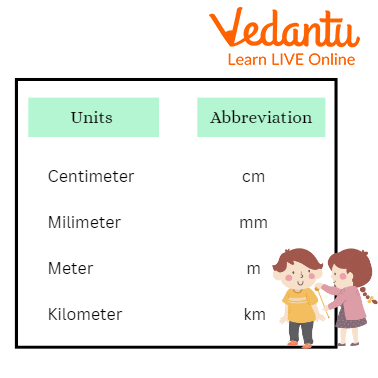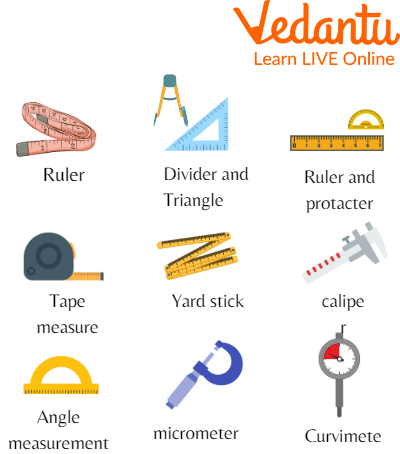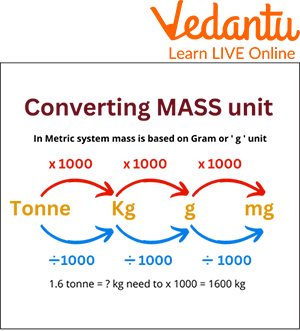Courses
Courses for Kids
Free study material
Free LIVE classes
More

# Smaller Units of LengthLIVE
Join Vedantu’s FREE Mastercalss

## An Overview of Units of Length

A unit of length is primarily used in the mathematical world. Measuring length means measurement of the length of any object with the help of measuring tools like a ruler, measuring tape, etc. For example, the length of a pencil can be measured in inches using a ruler. The height of students in a class can be measured using a feet scale. There are units of length like inches, centimetres, metres, feet, etc.

To convert larger units to smaller units, we multiply, and vice versa, we divide. This is a very simple process to convert larger units to smaller units. Another process of doing this, i.e., to convert a bigger unit to a smaller unit, we shift the decimal point to the right. Interestingly, this process is very commonly used in our daily lives.

## Units for Measuring Length

To measure length, there are different units. The bigger unit of measuring length is known to be kilometres. On the other hand, to measure smaller units of length, we use decimeters, centimetres, and millimetres. The standard unit of length is “metre”, which is written as ‘m’.

Normally, a metre length is divided into 100 equal parts. Interestingly, each of these parts is named centimetres, known as ‘cm’. Moreover, we could say 1 m = 100 cm and 100 cm = 1 m. For the bigger unit, kilometres are used, which equals 1000 metres, and kilometres are written as ‘km’ in short. We could say that 1 kilometre = 1000 metres and 1000 m = 1 km.Units of Measuring Length

Below is a table that talks of equivalents of different units of length.

 Units of Length Equivalent length 1 km 10 hectometers (hm) = 1000 m 1 hm 10 decathletes (dcm) = 100 m 1 dcm 10 metres 1 m 10 decimeters (dm) = 100 cm = 1000 mm 1 cm 10 millimetres (mm)

To convert kilometres into metres, one needs to multiply 1000. To convert metres into kilometres, one needs to divide it by 1000.

## Tools to Measure Length

To measure the length of any object or article, we require the right tool. If the right tool is not provided, then there might be a change in the result. In the same way, some important tools are required to measure length. These tools are used to measure both long and short lengths.

Some tools to measure length are as follows:-

• Ruler

• Tapes

• Feet/Foot

• Yardsticks

• metre sticks

• Calliper

• Micrometre

• Roll Meter

Some tools like the roll metre, calliper, metre sticks, and feet/foot are all used to measure long lengths. On the other hand, the smaller lengths are measured using a ruler, micrometre, calliper, and many more.Tools for Measuring Length

## Smaller Units of Mass

Many of you might have heard the word ‘mass’. It is the dimensionless quantity that represents the amount of matter in a particle. In the International System, the standard unit of mass is the kilogram (kg).

• The two smaller units of mass measurement are kilogram and gram. Interestingly, a milligram is the smallest unit of mass. The prefix ‘kilo’ literally means a thousand on the other hand, ‘milli’ means one-thousandths. The basic unit of mass is the gram and is used in many parts of the world.

• The equivalence of different units of mass is given below:

1 kilogram (kg) = 1,000 grams (g)

1 milligram (mg) = 0.001 gram (g)

• Here, to convert a bigger unit to a smaller unit, we multiply it by a power of ten. This will further help the decimal move to the right. On the other hand, to convert grams to milligrams, one needs to multiply it by 1000.

• In the case of mass, these were some ways to convert bigger units to smaller units.

• Now, to convert smaller units to larger units, one needs to divide to the power of ten. This will further help to move the decimal to the left side.

1 mg ÷ 1,000 = 0.001 g

1 g ÷ 1,000 = 0.001 kgConverting Mass to Other Units Process

## Solved Examples

Here are some simple examples to convert a unit of mass or length.

1. Change 7165 kg into quintals.

Ans: We need to calculate 7165 =? Quintals

Since 100 kg = 1 quintal

So, 7165 kg ÷ 100 = 71 quintals 65 kg

Therefore, 7165 kg equals 71 quintals 65 kg.

2. Change 345 cm into m.

Ans: As we need to calculate,

345 cm =? m

Since 1 m = 100 cm

So, 345 cm = 345 ÷ 100 = 3.45 m

Therefore, the result of conversion is 3.45 m.

## Practice Questions

1. Fill in the blank:

To convert a larger unit to a smaller unit, we _________.

Ans: Multiply it by powers of 10 or shift the decimal point to the right.

2. Convert 45.65 kg to a gram.

Ans: 45650 g.

3. Change 1000 centimetres to metres.

Ans: 10.

## Summary

Mathematics is an interesting subject to study. The calculation and conversion of the metric system make it more interesting for kids. Now, in this article, we are clear on how to convert larger units to smaller units, and smaller into bigger, two smaller units of mass, and smaller units of length. Here, we understand that to convert a larger value to a smaller one, we multiply it by the powers of 10. Some solved examples are also given that illustrate the method of conversion more deeply.

Last updated date: 29th Sep 2023
Total views: 96.9k
Views today: 2.96k

## FAQs on Smaller Units of Length

1. Which is the smallest unit of grams?

There are many units of measurement when it comes to mass. But, the smallest unit of grams is one picogram (pg) which is equal to 0.000000000001 gram.

2. What is the meaning of the unit of scale?

A unit of scale is nothing but a ratio. The ratio is used to compare the dimension of an actual object to the dimension of a scale in a drawing or any model.

3. What are the four types of measurement scales?

The four types of measurement scales are the following:

• Nominal Scale

• Ordinal Scale

• Interval Scale

• Ratio Scale